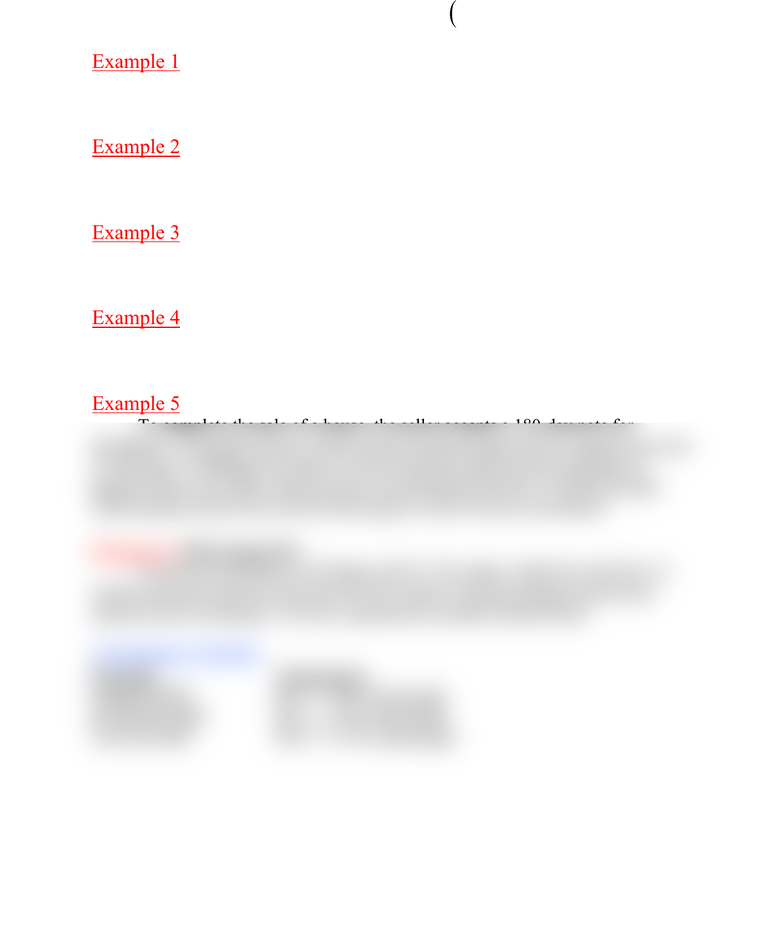# MT 1123 Lecture Notes - Interest, 18 Months

6 views1 pagesMTH 124 Section 3.1
I
Prt=
(
)
1
A
PI PPrt P rt=+=+ = +
Example 1
Find the total interest paid on a loan of \$500 at 8% simple interest over 2
years.
Example 2
You borrow \$3,000 at 9% simple interest for 4 years. How much will you
repay at the end of the 4 years?
Example 3
Find the total amount due on a \$200 loan at 7.5% simple interest over 18
months.
Example 4
You wish to deposit money into the bank at 9% simple interest and have
\$5,000 at the end of 5 years. How much should you deposit?
Example 5 (#50 on page 134)
To complete the sale of a house, the seller accepts a 180-day note for
\$10,000 at 7% simple interest. (Both interest and principal will be repaid at the end
of 180 days.) Wishing to be able to use the money sooner for the purchase of
another house, the seller sells the note to a third party for \$10,124 after 60 days.
What annual interest rate will the third party receive for the investment?
Example 6 (#52 on page 134)
An investor purchases 450 shares at \$21.40 a share, holds the stock for 26
weeks, and then sells the stock for \$24.60 a share. Find the annual interest rate
earned on the investment. Use the commission schedule found below.
Commission Schedule
Principal Commission
Under \$3,000 \$25 + 1.8% of principal
\$3,000-\$10,000 \$37 + 1.4% of principal
Over \$10,000 \$107 + 0.7% of principal
Unlock document

This preview shows half of the first page of the document.
Unlock all 1 pages and 3 million more documents.

## Document Summary

Find the total interest paid on a loan of at 8% simple interest over 2. You borrow ,000 at 9% simple interest for 4 years. Find the total amount due on a loan at 7. 5% simple interest over 18. You wish to deposit money into the bank at 9% simple interest and have. To complete the sale of a house, the seller accepts a 180-day note for. ,000 at 7% simple interest. (both interest and principal will be repaid at the end of 180 days. ) Wishing to be able to use the money sooner for the purchase of another house, the seller sells the note to a third party for ,124 after 60 days. Example 6 (#52 on page 134) weeks, and then sells the stock for . 60 a share. Find the annual interest rate earned on the investment.

## Get access